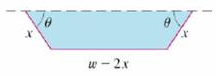Chapter 14, Problem 3P

Chapter
Section
Textbook Problem

A long piece of galvanized sheet metal with width w is to be bent into a symmetric form with three straight sides to make a rain gutter. A cross-section is shown in the figure.(a) Determine the dimensions that allow the maximum possible flow; that is, find the dimensions that give the maximum possible cross-sectional area.(b) Would it be better to bend the moral into a gutter with a semicircular cross-section?(a)

To determine

To find: The dimensions for the maximum cross sectional area which gives maximum flow for given cross sectional area.

Explanation

Given:

The rain gutter having width (w) and three straight sides is shown below in figure 1.

Calculation:

The area of the rain gutter is calculated using the area of trapezoid (A) as,

A=12h(b1+b2)

Here, height of the trapezoid is h and the upper and lower breadth of the trapezoid are b1 and b2 , respectively.

The cross section area of the rain gutter is the function of A(x,θ)=12h(b1+b2) .

Substitute x(sinθ) for h , (w2x) for b1 and w2x+2xcosθ for b2 ,

A(x,θ)=12(xsinθ)[(w2x)+(w2x+2xcosθ)]=12(xsinθ)(w2x+w2x+2xcosθ)=12(xsinθ)(2w4x+2xcosθ)=122(xsinθ)(w2x+xcosθ)

=(xsinθ)(w2x+xcosθ)A(x,θ)=wxsinθ2x2sinθ+x2sinθcosθ (1)

Here, 0<x12w and 0<θπ2

To find the critical point, differentiate the equation (1) with respect to x .

Equate the above equation is equal to zero (0) .

wsinθ4xsinθ+2xsinθcosθ=0sinθ(w4x+2xcosθ)=0w4x+2xcosθ=02xcosθ=4xw

cosθ=4xw2xcosθ=4x2xw2xcosθ=2w2x

Similarly, differentiate the equation (1) with respect to θ .

By chain rule of differentiation (ddx(u.v)=udvdx+vdudx) ,

Apply the formula (cos2θsin2θ=2cos2θ1) ,

Substitute 2w2x for cosθ ,

Substitute 0 for dAdθ ,

3x2wx=0x(3x

(b)

To determine

To find: Whether it would be better to bend the metal into a rain gutter with semicircular cross section.

Still sussing out bartleby?

Check out a sample textbook solution.

See a sample solution

The Solution to Your Study Problems

Bartleby provides explanations to thousands of textbook problems written by our experts, many with advanced degrees!

Get Started

Find more solutions based on key concepts